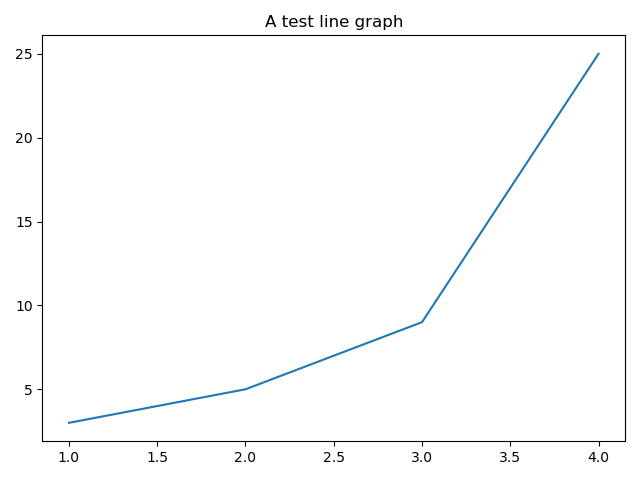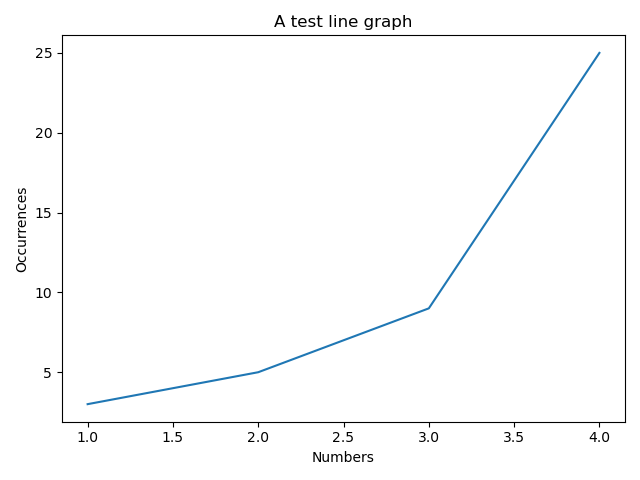# # The Object-Oriented Approach with matplotlib

Up until now we've been using the very popular approach of having a "currently active figure" and "currently active axes" where we draw plots:

``````import matplotlib.pyplot as plt

plt.figure()
plt.plot([1, 2, 3, 4], [3, 5, 9, 25])
plt.show()
``````

However, if you're developing a larger matplotlib application, rather than just small scripts and using it interactively, the object-oriented approach can help make your code more explicit.

This is what that same code looks like, using the object-oriented approach:

``````import matplotlib.pyplot as plt

figure = plt.figure()
axes.plot([1, 2, 3, 4], [3, 5, 9, 25])
plt.show()
``````

Now we're still using `plt.figure()`, but this time we're assigning its return value to a variable (a `Figure` object).

We're then using that object to interact with the figure. Here we're using `add_subplot()` to create a new space in the figure for our axes. It returns an `Axes` object, onto which we can plot our data using `.plot()`.

At the end, we use the `plt` library to call `.show()`.

In object-oriented code you'll sometimes see `figure.show()` instead, so what are the differences between `plt.show()` and `figure.show()`?

Since we're running this as a Python script, we want the graph to be displayed in its own window, with the appropriate controls to manipulate the graph and adjust its settings. We use `plt.show()` because it does all that for us.

However, if you wanted to embed the graph in a GUI application, a Jupyter notebook, or even the PyCharm Sci View, you could use `figure.show()` instead.

Almost everything we'll do with the object-oriented approach, you can do with the function-based approach too! However, I prefer the object-oriented approach because I feel the code it produces is clearer.

## # Setting the `Axes` title

Every set of axes can have a title displayed above them. We can use `.set_title()` on the `Axes` object to achieve this:

``````import matplotlib.pyplot as plt

figure = plt.figure()
axes.set_title("A test line graph")
axes.plot([1, 2, 3, 4], [3, 5, 9, 25])
plt.show()
``````## # Setting the x and y labels

Similarly, we can set x and y labels if we want to be more specific with the data they represent. Here we'll set the x axis label to Numbers and the y axis label to Occurrences:

``````import matplotlib.pyplot as plt

figure = plt.figure()